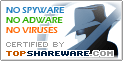# BreakTru Percent 6.2

SNAPSHOT

Find percentage of any number.

Windows AllPlatform :
\$0Price :
5.02 MBFile Size :
ScreenshotScreenshot :Popularity :Calculate the percentage of any number out of any number. Add percentage to an amount or calculate a discount percentage. For tip calculation. Figure the selling price after a discount was applied.

• Math Solver II
An advance scientific calculator with a step-by-step solution system, and the ability to plot one or more expressions.
• InnoCalculator
Multipurpose and comprehensive calculator
• free 642-902 exam demo
latest 642-902 exam demo offered by Certidea
• Math Expression Generator
An application to generates math expressions.
• Fret Specs
Fret Specs fret calculator for the stringed instrument craftsman and luthier
• Galactic Geometry 3D
3D game for learning volume and surface area.
• AutoSignal
Scientists and engineers can perform complex signal analysis without programming by selecting menu items that determine how the computer will analyze and present data.
• Scuba Diving Calculators
Scuba Diving Calculators
• Visual Complex
Visual Complex is a graph software to create graph of complex function.
• NLREG
NLREG performs nonlinear regression and curve fitting